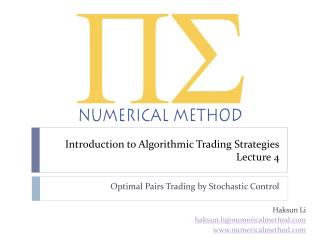Download PresentationIntroduction to Algorithmic Trading Strategies Lecture 4

# Introduction to Algorithmic Trading Strategies Lecture 4 - PowerPoint PPT PresentationDownload Presentation## Introduction to Algorithmic Trading Strategies Lecture 4

- - - - - - - - - - - - - - - - - - - - - - - - - - - E N D - - - - - - - - - - - - - - - - - - - - - - - - - - -
##### Presentation Transcript

1. Introduction to Algorithmic Trading StrategiesLecture 4 Optimal Pairs Trading by Stochastic Control Haksun Li haksun.li@numericalmethod.com www.numericalmethod.com

2. Outline • Problem formulation • Ito’s lemma • Dynamic programming • Hamilton-Jacobi-Bellman equation • Riccatiequation • Integrating factor

3. Reference • Optimal Pairs Trading: A Stochastic Control Approach. Mudchanatongsuk, S., Primbs, J.A., Wong, W. Dept. of Manage. Sci. & Eng., Stanford Univ., Stanford, CA.

4. Basket Creation vs. Trading • In lecture 3, we discussed a few ways to construct a mean-reverting basket. • In this and the next lectures, we discuss how to trade a mean-reverting asset, if such exists.

5. Stochastic Control • We model the difference between the log-returns of two assets as an Ornstein-Uhlenbeckprocess. • We compute the optimal position to take as a function of the deviation from the equilibrium. • This is done by solving the corresponding the Hamilton-Jacobi-Bellman equation.

6. Formulation • Assume a risk free asset, which satisfies • Assume two assets, and . • Assumefollows a geometric Brownian motion. • is the spread between the two assets.

7. Ornstein-UhlenbeckProcess • We assume the spread, the basket that we want to trade, follows a mean-reverting process. • is the long term equilibrium to which the spread reverts. • is the rate of reversion. It must be positive to ensure stability around the equilibrium value.

8. Instantaneous Correlation • Let denote the instantaneous correlation coefficient between and .

9. Univariate Ito’s Lemma • Assume • is twice differentiable of two real variables • We have

10. Log example • For G.B.M., ,

11. Multivariate Ito’s Lemma • Assume • is a vector Ito process • is twice differentiable • We have

12. Multivariate Example

13. What is the Dynamic of Asset At?

14. Dynamic of Asset At

15. Notations • : the value of a self-financing pairs trading portfolio • :the portfolio weight for stock A • :the portfolio weight for stock B

16. Self-Financing Portfolio Dynamic

17. Power Utility • Investor preference:

18. Problem Formulation • , s.t., • , • Note that we simplify GBM to BM of , and remove some constants.

19. Dynamic Programming • Consider a stage problem to minimize (or maximize) the accumulated costs over a system path. • Cost = s11 S21 2 5 3 3 s0 s12 s3 S22 4 1 5 5 s23 3 s12 time t = 1 t = 0 t = 2

20. Dynamic Programming Formulation • State change: • : time • : state • : control decision selected at time • : a random noise • Cost: • Objective: minimize (maximize) the expected cost. • We need to take expectation to account for the noise, .

21. Principle of Optimality • Let be an optimal policy for the basic problem, and assume that when using , a give state occurs at time with positive probability. Consider the sub-problem whereby we are at at time and wish to minimize the “cost-to-go” from time to time . • Then the truncated policy is optimal for this sub-problem.

22. Dynamic Programming Algorithm • For every initial state , the optimal cost of the basic problem is equal to , given by the last step of the following algorithm, which proceeds backward in time from period to period

23. Value function • Terminal condition: • DP equation: • By Ito’s lemma:

24. Hamilton-Jacobi-Bellman Equation • Cancel on both LHS and RHS. • Divide by time discretization, . • Take limit as , hence Ito. • The optimal portfolio position is .

25. HJB for Our Portfolio Value

26. Taking Expectation • All disapper because of the expectation operator. • Only the deterministic terms remain. • Divide LHR and RHS by .

27. Dynamic Programming Solution • Solve for the cost-to-go function, . • Assume that the optimal policy is .

28. First Order Condition • Differentiate w.r.t. . • In order to determine the optimal position, , we need to solve for to get , , and .

29. The Partial Differential Equation (1)

30. The Partial Differential Equation (2)

31. Dis-equilibrium • Let . Rewrite: • Multiply by .

32. Simplification • Note that • The PDE becomes

33. The Partial Differential Equation (3)

34. Ansatz for G

35. Another PDE (1) • Divide by .

36. Ansatz for

37. Boundary Conditions

38. Yet Another PDE (1) • Divide by .

39. Yet Another PDE (2)

40. Expansion in

41. Grouping in

42. The Three PDE’s (1)

43. PDE in

44. PDE in,

45. PDE in,,

46. Riccati Equation • A Riccati equation is any ordinary differential equation that is quadratic in the unknown function.

47. Solving a Riccati Equation by Integration • Suppose a particular solution, , can be found. • is the general solution, subject to some boundary condition.

48. Particular Solution • Either or is a particular solution to the ODE. This can be verified by mere substitution.

49. Substitution • Suppose . goes to 0 by the definition of

50. Solving • 1st order linear ODE